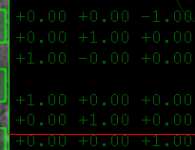# Quaterinions and autopilot questions

#### Topper

Donator
Hello after a few years I started to continue some projects for Orbiter.
But I'm a bit out from my thoughts years ago and cannot remember all the staff so I have some questions:

First of all I want to remove some "Bug" I did using quaternions so I guess I builded them wrong and corrected somhow on another position in the code...
So I have two methods to create a quaternion and my question is which one is correct:

First method is this (currently active):
Code:
        void yprToQuat(VECTOR3 ypr)
{
// Create quaternian from setpoints
double c1 = cos((ypr.z - PI05)/2);
double s1 = sin((ypr.z - PI05)/2);
double c2 = cos(ypr.y/2);
double s2 = sin(ypr.y/2);
double c3 = cos(-ypr.x/2);
double s3 = sin(-ypr.x/2);
double c1c2 = c1*c2;
double s1s2 = s1*s2;

w = c1c2*c3 - s1s2*s3;
x = c1c2*s3 + s1s2*c3;
y = s1*c2*c3 + c1*s2*s3;
z = c1*s2*c3 - s1*c2*s3;
}
second method is this (seen at wikipedia):
Code:
        void set(double yaw, double pitch, double roll)
{
// Abbreviations for the various angular functions
double cy = cos(yaw * 0.5);
double sy = sin(yaw * 0.5);
double cp = cos(pitch * 0.5);
double sp = sin(pitch * 0.5);
double cr = cos(roll * 0.5);
double sr = sin(roll * 0.5);

//Quaternion q;
this->w = cr * cp * cy + sr * sp * sy;
this->x = sr * cp * cy - cr * sp * sy;
this->y = cr * sp * cy + sr * cp * sy;
this->z = cr * cp * sy - sr * sp * cy;
}
To get the rotation matrix from the quaternion, I used this method (for both):
Code:
        MATRIX3 getMatrix()
{
// create matrix from the set point quaternions
double sqw = w*w;
double sqx = x*x;
double sqy = y*y;
double sqz = z*z;

// invers (inverse square length) is only required if quaternion is not already normalised
double invs = 1 / (sqx + sqy + sqz + sqw);

MATRIX3 mq;
mq.m11 = ( sqx - sqy - sqz + sqw)*invs ; // since sqw + sqx + sqy + sqz =1/invs*invs
mq.m22 = (-sqx + sqy - sqz + sqw)*invs ;
mq.m33 = (-sqx - sqy + sqz + sqw)*invs ;

double tmp1 = x*y;
double tmp2 = z*w;
mq.m21 = 2.0 * (tmp1 + tmp2)*invs ;
mq.m12 = 2.0 * (tmp1 - tmp2)*invs ;

tmp1 = x*z;
tmp2 = y*w;
mq.m31 = 2.0 * (tmp1 - tmp2)*invs ;
mq.m13 = 2.0 * (tmp1 + tmp2)*invs ;
tmp1 = y*z;
tmp2 = x*w;
mq.m32 = 2.0 * (tmp1 + tmp2)*invs ;
mq.m23 = 2.0 * (tmp1 - tmp2)*invs ;
return mq;
}

And I got this matrices which are different, when I set yaw pitch and roll to 0.So am I correct when I assume that the " void yprToQuat(VECTOR3 ypr)" method is wrong somehow?
If this is the case more question will follow I guess ?